# 7th Grade - Area of Trapezoids

## Introduction

• A trapezoid or trapezium is a quadrilateral that has only one pair of opposite sides parallel.
• These parallel sides are called bases and the remaining non-parallel sides are called legs.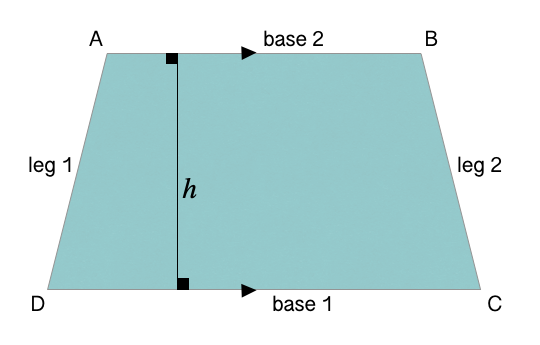• There are two types of trapezoids:

1. Right Trapezoids: If one leg of a trapezoid makes a pair of right angles with the two bases, it is called a right trapezoid.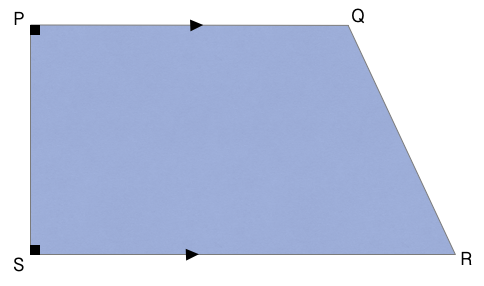2. Isosceles Trapezoids: If the legs of a trapezoid are equal, it is called an isosceles trapezoid.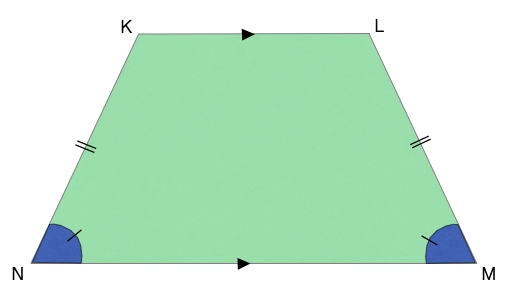## Area of Trapezoids

• The area of a trapezoid is the region enclosed by its four sides.
• The area of a trapezoid depends upon the measure of its bases and height.
• If we know the length of the bases, and the height of a trapezium, we can determine its area using the formula mentioned below.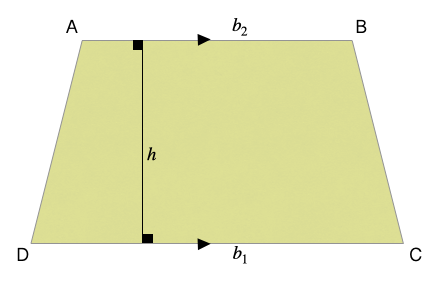, where  are the length of bases, and $h=$height.

• The perimeter of a trapezoid is the sum of the length of all its sides.

## Some Solved Examples

Question 1: Find the area of the trapezium shown in the figure below.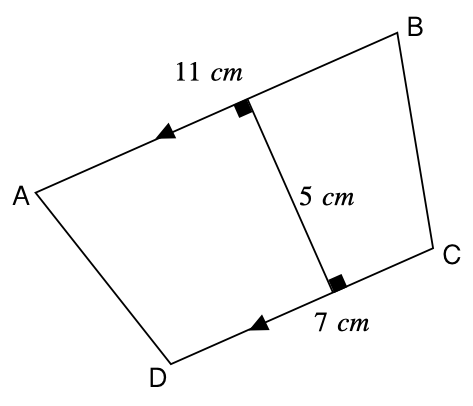Solution: $Are{a}_{trapezoid}=\frac{{b}_{1}+{b}_{2}}{2}×h$$=\frac{7+11}{2}×5$$=\frac{18}{2}×5$$=9×5$

Question 2: Find the area of the trapezium shown in the figure below.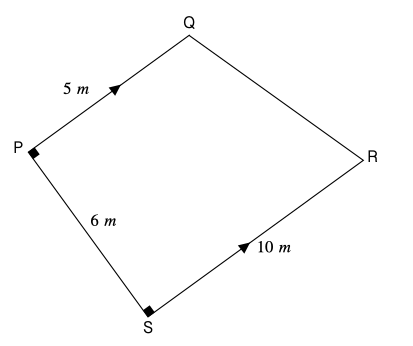Solution: $Are{a}_{trapezoid}=\frac{{b}_{1}+{b}_{2}}{2}×h$$=\frac{5+10}{2}×6$$=\frac{15}{2}×6$$=15×3$

## Cheat Sheet

• The legs of an isosceles trapezoid are equal.
• The perimeter of a trapezoid is the sum of all its sides.
• $Are{a}_{trapezoid}=\frac{{b}_{1}+{b}_{2}}{2}×h$, where ${b}_{1},{b}_{2}=$length of bases, and $h=$height of the trapezoid

## Blunder Areas

• To find the area of a trapezoid, correctly identify its bases and height first.
•  Note that the height of a trapezoid is perpendicular to both bases.